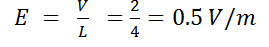# A potentiometer consists of a wire of length 4 m and resistance 10W. It is connected to a cell of emf 2V. The potential gradient of wire is         (a)    0.5 V/m         (b)    2 V/m         (c)    5 V/m         (d)    10 V/mAditi Chauhan
9 years ago

(a)

Potential across wire = 2V

Length of wire = 4 m?
4 years ago
As we know I max. Is E/R,
I= E/R  = 2V/10 = 1/5 Ampere
And voltage is I max.  × Resistance ÷ Length ( which is 4m)
V= I × R/L   =  1×10 / 20 = 0.05V/m